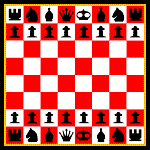## CryptogramsIn Cryptograms you are given sentences in which one set of letters is substituted for another. You can break the code by watching for the frequency of certain letters. For example "MHP" could be "the" and "MHEM" would be "that." Cryptograms are not easy--so if you need help in getting started, click on the "Hint" link next to each puzzle. You might want to print out this page and solve these on paper.

 _ _ _ _ _ _ _ _ _ _ _ _ _ _ _ _ _ _ _ _ _ _ _ _ _ _ Q C F E E X H K O F V O M F B P B K O C E V U W K X _ _ _ _ _ _ _ _ _ _ _ _ _ _ _ _ _ _ _ _ _ _ _ _ _ _ _ _ _ _ H C S E M P X D S O V H. F B V O R V N N B P M F K E W V I B

 _ ' _ _ _ _ _ _ _ _ _ _ _ _ _ _ _ _ _ _ N ' R B I C C A W N B J C I T N B P G B _ _ _ _ _ _ ' _ _ _ _ _ _ _ _ _ _ _ _ _ _ _ _ _ N R G J D. N ' R C AW N B J C I L D R W E D S Y

 _ _ _ _ _ _ _ _ _ _ _ _ _ _ _ _ _ _ _ _ _ _ _ C R U T G C G J U N T E N L B O J F H I P C Q. _ _ _ _ _ _ _ _ _ _ _ _ _ _ _ _ _ _ _ _ _ _ _ _ _ C Z J E J D I V U C U S E T P C V T J N N G T O Y T _ _ _ _ _ _ _ _ _ _ _ _ _ _ _ _ _ _ F T J V P L O E N L D T L U J D I P

 _ _ _ _ _ _ _ _ _ _ _ _ _ _ _ _ _ P H A P R S Q A B R P H H O J C T _ _ _ _ _ _ _ _ _ _ _ _ _ _ _ _ _ _ _ _ _ _ _, _ _ _ D E W B L H E L P R I P R I A B U O H N B C H, E J H _ _ _ _ _ _ _ _ _ _ _ _ _ _ _ _ _ E N H A B A B U O H U R F L E J C _ _ _ _ _ _ _ _ _ _ _ _ _ _, _ _ _ _ _ _ _ _ _ _ _ E N D E J R H O T W J L P D, I B E O I B X E R B L _ _ _ _ _ _ _ _. _ _ _ _ _ _ _ P L E J O W U R. A B H O J C T _ _ _ _ _ _ _ _ _ __ _ _. _ _ _ _ _ _ _ _ _ _ _ _ _. P L H A B J C H P W U H B D E J R H O T L P R I B O.

 _ _ _ _ _ _ _ _ _ _ _ _ _ _ _ _ _ _ _ N C S O L F C S O B H U P Q B I O A U _ _ _ _ _ _ _ _ _ _ _ _ _ _ _ _ _ _ A W A D L S P T N F K A A R R P O A _ _ _ _ _ _ _ _ _ _ _ _ _ _ ' _ _ _ _ _ _ _ _ _ _ U I F H D M I L S P T N F. P ' R R Q C S U P M A D _ _ _ _ _ _ _ _ _ _ _ _ _ _ _ _ _ _ _ _ P F I U H Q Q A U U K H R A W A S P S T _ _ _ _ _ _ _ _ _ _ P K J A T A F C H F _ _ _ _ _ _ _ _ _ _ _ _ _ _ _ _ _ _ _ _ C K N A D A J P F N C H F T A F F P S T _ _ _ _ _ _ _ _. P S I K P T N F.